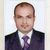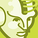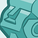# QlikView App Dev

Discussion Board for collaboration related to QlikView App Development.

Announcements
Our next Qlik Insider session will cover new key capabilities. Join us August 11th REGISTER TODAY
cancel
Showing results for
Did you mean:Creator II

## greater than equal to or less than equal to in set analysis expression

How to use greater than equal to or less than equal to in set analysis expression?

Give an example

1 Solution

Accepted SolutionsMaster III

Greater than or equal to

sum({<AMOUNT = {">=\$(vMin)"}>} AMOUNT)

Lesser than or equal to

sum({<AMOUNT = {"<=\$(vMax)"}>} AMOUNT)

Greater than or equal to and lesser than or equal to

sum({<AMOUNT = {">=\$(vMin)<=\$(vMax)"}>} AMOUNT)

count({<Date={"\$(= ‘>=‘ & ‘01/01/2010’ & ‘<=‘ & ‘31/12/2012’)"}>} Dim)

4 RepliesMaster III

Greater than or equal to

sum({<AMOUNT = {">=\$(vMin)"}>} AMOUNT)

Lesser than or equal to

sum({<AMOUNT = {"<=\$(vMax)"}>} AMOUNT)

Greater than or equal to and lesser than or equal to

sum({<AMOUNT = {">=\$(vMin)<=\$(vMax)"}>} AMOUNT)

count({<Date={"\$(= ‘>=‘ & ‘01/01/2010’ & ‘<=‘ & ‘31/12/2012’)"}>} Dim)MVP

See the below examples

For Greated then equals

For Less then equals

Or combination of both

But depends on the condition where you applyChampion

sum({<Datefield={">=\$(=Datefield)<=\$(=Datefield)"}>}  Amount)

or  greater than or equal to

sum({<Datefield={">=\$(=Datefield)"}>}  Amount)

or

less than or equal to

sum({<Datefield={"<=\$(=Datefield)"}>}  Amount)

hope this helpsContributor

Currently, this solution doesn't work.Community Browser